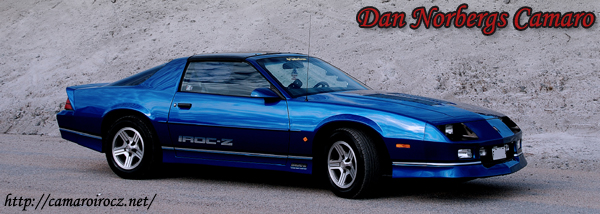..:: VIN DECODER ::..

1982-1984

 1 Country 1 = USA 2 = Canada 2 Manufacturer G = General Motors 3 Make 1 = Chevrolet 2 = Pontiac 4 Restraints A = Manual Belts B = Automatic Belts 5 Carline P = Sport Coupe, Z28  S = Berlinetta  S = Firebird  W = Trans Am X = Trans Am Special Edition 6 Body type 87 = 2 Door Coupe 7 Body type Used with postion six. 8 Engine F = 151ci, L4 (LQ8) 2 = 151ci, L4 (LQ9) 1 = 173ci, V6 (LC1) H = 305ci, V8 (LG4) G = 305ci, V8 (L69) 7 = 305ci, V8 (LU5) S = 305ci, V8 (LU5) 9 Check Digit The number produced when all numbers and characters(converted to numbers) are added up untill it is down to a single digit (ex. 12345=15=6) 10 Model Year C = 1982 D = 1983 E = 1984 11 Plant N = Norwood L = Van Nuys 12-17 Production Sequence This is a number assigned to the car during production

1985-1986

 1 Country 1 = USA 2 = Canada 2 Manufacturer G = General Motors 3 Make 1 = Chevrolet 2 = Pontiac 4 Carline F = F-body 5 Carline series P = Sport Coupe, Z28 S = Berlinetta S = Firebird W = Trans Am X = T/A Special Edition 6 Body type 87 = 2 door coupe 7 Body type Used with position six 8 Engine 2 = 151ci, L4 (LQ9) 1 = 173ci, V6 (LB8) S = 173ci, V6 (LB8) F = 305ci, V8 (LB9)[Tuned Port Injection] H = 305ci, V8 (LG4)[4BBL] G = 305ci, V8 (L69)[4BBL HO} 9 Check digit The number produced when all numbers and characters(converted to numbers) are added up untill it is down to a single digit (ex. 12345=15=6) 10 Model Year F = 1985 G = 1986 11 Assembly Plant N = Norwood L = Van Nuys 12-17 Production Sequence This is a number assigned to the car during production

1987-1992

 1 Country 1 = USA 2 = Canada 2 Manufacturer G = General Motors 3 Make 1 = Chevrolet 2 = Pontiac 4 Carline F = F-body 5 Carline series P = Camaro (all models) S = Firebird W = Trans Am 6 Body type 2 = 2 door coupe 3 = Convertible 7 Restraints 1 = Manual Belts 3 = Manual Belts, Drivers Air Bag 4 = Automatic belts 8 Engine S = 173ci, V6 (LB8)[1987-1989] T = 191ci, V6 (LH0)[1990-1992] H = 305ci, V8 (LG4)[4BBL] E = 305ci, V8 (LO3)[TBI][1988-1992] F = 305ci, V8 (LB9)[Tuned Port Injection] 8 = 350ci, V8 (L98)[Tuned Port Injection] 9 Check Digit The number produced when all numbers and characters(converted to numbers) are added up untill it is down to a single digit (ex. 12345=15=6) 10 Model Year H = 1987 J = 1988 K = 1989 L = 1990 M = 1991 N = 1992 11 Assembly Plant N = Norwood L = Van Nuys 12-17 Production Sequence This is a number assigned to the car during production

MENY

- Home
- Gästbok / Admin
- Länkar

- Back